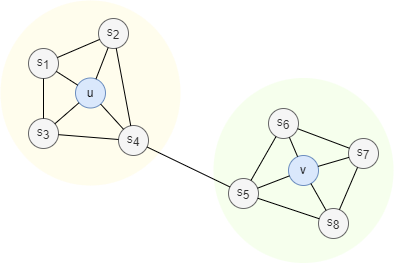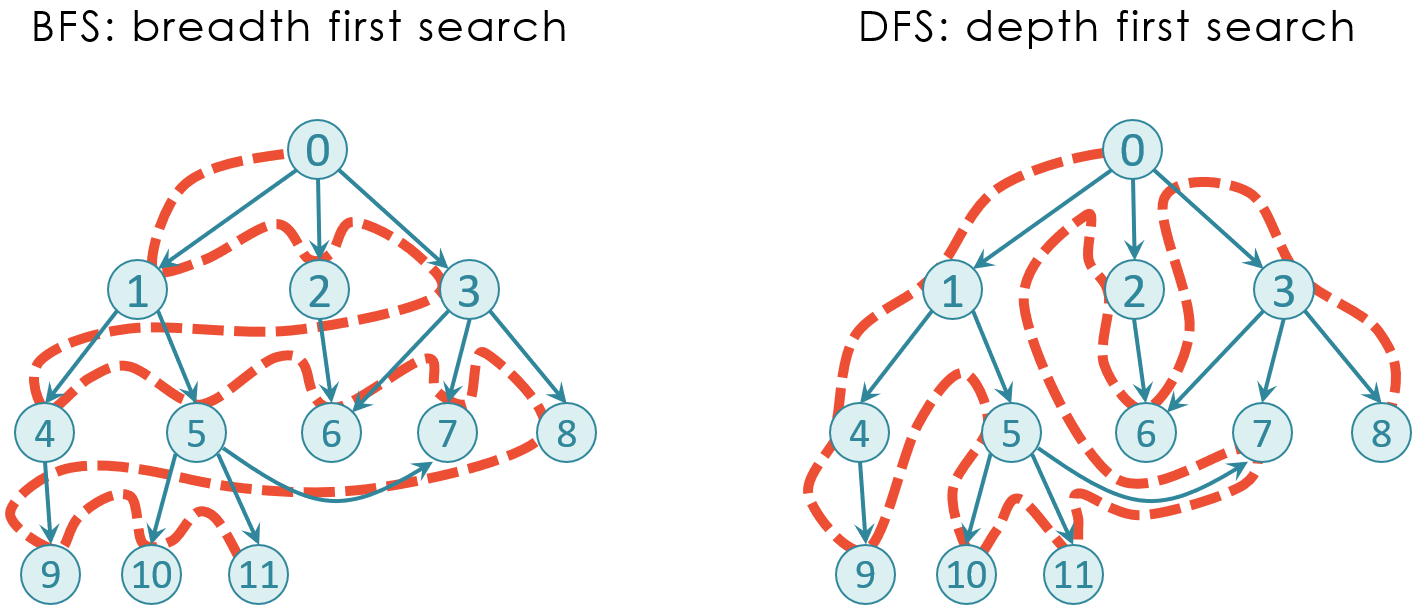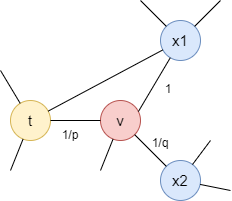# Change Nickname

Current Nickname:

Certifications

Certificate Issued at Valid until Serial No. File
Serial No. Valid until

Not having one? Apply now! >>>

Invoice

ProductName CreateTime ID Price File
ProductName CreateTime ID Price File

# Node2Vec

## Overview

Node2Vec graph embedding algorithm takes both the BFS and DFS neighborhood of node into consideration, node sequences are generated through a biased second-order random walk and sent to the Skip-gram model, which was originally proposed in Word2Vec algorithm, to train node embeddings. Node2Vec was proposed by A. Grover and J. Leskovec of Stanford University in 2016.

Related materials of the algorithm:

## Basic Concept

### Node Similarity

Nodes in network could be classified based on two kinds of similarities: homophily and structural equivalence. Homophily emphasizes the connections between nodes, while structural equivalence points at the local topology of nodes rather than connectivity. Nodes with structural equivalence or similarity could be far apart in the network.#### Homophily

Neighbors of a node often share similar features (properties) with the node, which is referred as homophily. Classically, in a social network, a user (node) and his friends (neighbors) are expected to have similar interests, age and so on. Nodes with homophily are usually found close to each other in the network. In the graph above, nodes u, s1, s2, s3 and s4 are in the same community or cluster and have homophily.

#### Structural Equivalence

In general, structural equivalence means that the neighborhood topology of two nodes is homogeneous or symmetrical. When considering structural equivalence, two things should be noted: First, complete structural equivalence in the real network is rare, so we tend to consider structural similarity instead. Second, the larger the neighborhood that is considered, the lower the structural similarity of the two nodes is. In the above graph, nodes u and v both act as hubs of their corresponding communities, thus they have a good degree of structural equivalence.

### BFS and DFS

In graph theory, Breadth First Search (BFS for short) refers to starting from a node and traversing adjacent nodes from near to far. It first traverses the neighbors of the previous layer before traversing the neighbors of the next layer. For example, K-Hop query in the graph is typical BFS.

Compared with the horizontal (breadth) first search of BFS, DFS (Depth First Search) is a vertical (depth) first search, which starts from the current node for a deep exploration until reaches the maximum limited search depth, only then it will return to the previous layer to continue the search. The search stops until a certain node or edge is found, or the whole graph is traversed.### Second-order Random Walk

In classic random walk, by default each edge has the same probability to be picked (as detailed in chapter Random Walk), and the next node chosen by the current node is not affected by previous visited nodes. The so-called Second-order Random Walk (or RW2 in short) refers to the random walk that takes the previous visited nodes into consideration when selecting the next node to visit.

### Node2Vec Random Walk

Node2Vec introduces two parameters `p` and `q` to control whether the walk is more BFS or DFS. Assuming a node walks from `t` to `v` and `v` has neighbor node `x`, the weight of the adjacent edges of `v` are influenced by the shortest distance `d` between `t` and `x`, and it is done with the parameters `p` and `q`:

• When `d = 0` (i.e. `x` equals to `t`), the corresponding edge weight is multiplied with `1/p`. `x` equals to `t` means the node returns to the previous visited node, thus `p` is also called the `return` parameter; the larger `p` is, the smaller the probability of returning.
• When `d = 1` (i.e `x` equals to `x1` in the graph below), the corresponding edge weight is not influenced. Both `x1` and `t` are the 1-hop neighbors of `v`, node walking to `x1` means it does not walk far away.
• When `d = 2` (i.e `x` equals to `x2` in the graph below), the corresponding edge weight is multiplied with `1/q`. Node walking to `x2` means it moves far away, thus `q` is also called the `in-out` parameter; `q > 1` means node tends to walk at the same level, `q < 1` means tend to walk far away.The probability of the current node walking along each adjacent edge is then obtained after normalizing the influenced weight of each edge.

When leaning to walk in the BFS neighborhood, the final embedding result reflects more of the structural equivalence of nodes, because the walking process is exploring the environment (topology) around the node. When leaning to walk in the DFS neighborhood, the final embedding result reflects more the homophily of nodes, because the walking process is exploring the environment within the community of the node.

### Node Embeddings

In 2014. B. Perozzi et al. proposed the DeepWalk graph embedding algorithm, they first applied the deep learning technology which was widely used in NLP field to network analytics. DeepWalk generalizes language modeling to the process of exploring graph through random walks, it treats the walk sequences as special kind of phrases. Similarly, Node2Vec uses the Skip-gram language model and SGD to train walk sequences to get the node embeddings in vector space and optimizes the training process with negative sampling and so on.

## Special Case

### Lonely Node, Disconnected Graph

Lonely node has no adjacent node, hence it cannot walk to other nodes; lonely node can only walk along its self-loop edge if there is one. The walk paths of non-lonely nodes will not have any lonely node.

Node only walks within its own connected component.

### Self-loop Edge

Node may walk along its self-loop edge.

### Directed Edge

For directed edges, Node2Vec algorithm ignores the direction of edges but calculates them as undirected edges.

## Command and Configuration

• Command：`algo(node2vec)`
• Configurations for the parameter `params()`:
Name Type
Default
Specification
Description
ids / uuids []`_id` / []`_uuid` / / IDs or UUIDs of nodes to start the walk; all nodes to be selected if not set
walk_length int 1 >=1 Depth of each walk, i.e., the number of nodes walking through
walk_num int 1 >=1 Number of walks
p float 1 >0 `return` parameter; the larger the value, the smaller the probability of returning
q float 1 >0 `in-out` parameter that represents the probability of being to walk far away; >1 means tend to walk at the same level, >1 means tend to walk far away
edge_schema_property []`@<schema>?.<property>` / Numeric edge property, LTE needed Edge weight property/properties, schema can be either carried or not; nodes only walk along edges with the specified properties and the probability of passing through these edges is proportional to the edge weight; if edge has multiple specified properties, the edge weight is the sum of these property values; the weight of all edges is 1 if not set
window_size int / >=1 Size of the sliding window, to sample `window_size` nodes on left and `window_size` nodes on right
dimension int / >=1 Dimension of node embedding vector
learning_rate float / (0,1) Initial learning rate, it decreases until reaches `min_learning_rate` with increase in training iterations
min_learning_rate float / (0,`learning_rate`) Minimum learning rate
min_frequency int / >=0 Minimum number of occurrences of a node in the walk sequences to be included in the model, <=1 means to keep all nodes
sub_sample_alpha float / / Threshold for sub sampling, <=0 means no sub sampling
resolution int / >=1 Such as 10, 100
neg_num int / >=0 Number of negative samples, it is suggested 0~10
loop_num int / >=1 Number of training iterations (epochs)
buffer_size int 1000 / Number of random walks to complete before starting training, <0 means to wait for all random walks to finish
limit int -1 >=-1 Number of results to return; return all results if sets to -1 or not set

Example: Run Node2Vec in the whole graph

``````algo(node2vec).params({
walk_length: 3,
walk_num: 2,
p: 1,
q: 1,
window_size: 5,
dimension: 5,
learning_rate: 0.025,
min_learning_rate: 0.00025,
min_frequency: -1,
sub_sample_alpha: -1,
resolution: 2,
neg_num: 0,
loop_num: 2,
limit: -1
}) as results
return results
``````

## Algorithm Execution

#### 1. File Writeback

Configuration Data in Each Row
filename `_id`,`embedding_result`

#### 2. Property Writeback

Not supported by this algorithm.

#### 3. Statistics Writeback

This algorithm has no statistics.

### Direct Return

Alias Ordinal Type
Description
Column Name
0 []perNode Array of UUIDs and embeddings of nodes `_uuid`, `embedding_result`

### Streaming Return

Alias Ordinal Type
Description
Column Name
0 []perNode Array of UUIDs and embeddings of nodes `_uuid`, `embedding_result`

### Real-time Statistics

This algorithm has no statistics.